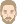FLECAR
WUFI UserPosts: 5
Joined: Fri Jan 03, 2020 6:03 am -1100

Hello,

When I simulate a with a epw climate file the "atmospheric counterradation" displayed by WUFI interface in the outdoor climate menu corresponds to the field N12-Horizontal Infrared Radiation Intensity (https://bigladdersoftware.com/epx/docs/ ... onary.html).
example : on 01/01 at 2pm, WUFI diplays a "atmospheric counterradation" of 419W/m2

However, when I checked the ASCII file with the different components of the radiation, I find totally different value in the column "atmospheric counterradation"
example : on 01/01 at 2pm, WUFI ASCII informs the following values:

So my questions are:
- What are the values considered by WUFI when calculating radiative exchanges with the explicit radiation mode (the one displayed on the interface or the ones in the ASCII files)?
- How the radiative counterradiation components in ASCII files are calculated from the information of the epw climate file?
AttachmentsWUFI_Interface_atmospheric counterradiation_1Jan.PNG (13.4 KiB) Viewed 1884 timesWUFI_Interface_atmospheric counterradiation.PNG (17.43 KiB) Viewed 1884 timesWUFI_ASCII_atmospheric counterradiation_1Jan.PNG (61.19 KiB) Viewed 1884 times

ThomasPosts: 294
Joined: Sun Jun 19, 2005 10:33 pm -1100

Hello FLECAR,

the "Counterradiation" column in the ASCII output shows the total long-wave radiation incident on the surface. It is the sum of the following radiation components:

* the long-wave radiation from the sky, as incident on the simulated surface: g * ILAH
where ILAH is the long-wave atmospheric irradiance on a horizontal plane and g is the view factor between the simulated surface and the sky. For a horizontal simulated surface (e.g. a flat roof) g is 1, for a vertical simulated surface (e.g. a wall) g is 0.5.

* the long-wave radiation emitted by the sky and then reflected by the ground, as incident on the simulated surface: (1-g) * (1-e) * ILAH
where ILAH is the long-wave atmospheric irradiance on a horizontal plane, (1-e) is the long-wave reflectivity of the ground (e being the long-wave emissivity), and (1-g) is the view factor between the simulated surface and the ground (g being the view factor towards the sky, see above)

* the long-wave radiation thermally emitted by the ground, as incident on the simulated surface: (1-g) * ILTH
where ILTH is the thermal emission by the ground and (1-g) is the view factor between the simulated surface and the ground.

ILAH is usually provided by the weather file. ILTH is sometimes provided by the weather file (depending on the weather file format and the availability of measured radiation data). If ILTH is not provided by the file, WUFI estimates it by assuming that the ground surface temperature is the same as the air temperature:

* ILTH = e * 5.67e-8 * (t_air+273.15)^4
where e is the long-wave emissivity of the ground surface.

Apparently you did the simulation for a vertical wall, g = (1-g) = 0.5, and you used the default ground emissivity e = 0.9. This results in the following radiation components:

* the long-wave radiation from the sky as incident on the vertical surface is 0.5 * 419 = 209.5 W/m2, identical with the value shown in the ASCII output.
* the ground-reflected long-wave radiation as incident on the vertical surface is (1-0.5) * (1 - 0.9) * 419 = 20.95 W/m2, identical with the value shown in the ASCII output.
* the thermal emission of the ground as incident on the vertical surface is (1-0.5) * 0.9 * 5.67e-8 * (28.4 + 273.15)^4 = 210.976 W/m2, identical with the value shown in the ASCII output. 28.4 °C is the air temperature for that hour.

The total long-wave radiation incident on the simulated wall is the sum of all these components:

441.426 = 209.5 + 210.976 + 20.95Kind regards,
Thomas

FLECAR
WUFI UserPosts: 5
Joined: Fri Jan 03, 2020 6:03 am -1100